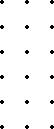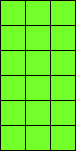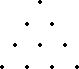# Definitions 15–19

15. A number is said to multiply a number when the latter is added as many times as there are units in the former.

16. And, when two numbers having multiplied one another make some number, the number so produced be called plane, and its sides are the numbers which have multiplied one another.

17. And, when three numbers having multiplied one another make some number, the number so produced be called solid, and its sides are the numbers which have multiplied one another.

18. A square number is equal multiplied by equal, or a number which is contained by two equal numbers.

19. And a cube is equal multiplied by equal and again by equal, or a number which is contained by three equal numbers.

## Guide

Notice that Euclid doesn’t define addition and subtraction. For an example where addition of numbers appears, see VII.28. Formal definitions for them could be given, but those operations are assumed to be understood in the same way that any number a is a multiple of units u.

Multiplication and proportion are formally defined, and proportion is defined next in VII.Def.20.

#### On Definition 15

Definition 15 defines multiplication in terms of addition as a kind of composition. For instance, to multiply a = 4u and b = 5u, since a consists of 4 units, add 4 b’s together, that is, add 5u, 5u, 5u, and 5u.

Note that Euclid did not think of addition as a binary operation, but as an operation with any number of arguments. Definition 15 uses the informal operation of addition to define the formal operation of multiplication.

The first proposition on multiplication is VII.16 which says multiplication is commutative. For our example, that would say a times b equals b times a. Since b = 5u and a = 4u, therefore b times a requires adding 4u, 4u, 4u, 4u, and 4u.

#### Figurate numbers

Although Euclid never displays numbers except as lines, the Pythagoreans before him evidently did, that is, they displayed numbers as figures. The figures were in various shapes, such as triangles, squares, and so forth. Definitions 16 through 19 deal with figurate numbers, but without the figures.Euclid defines a plane number as a number which is the product of two numbers. Remember that for Euclid, 1 is the unit, not a number, so a prime number is not a plane number, even though it is a product of 1 and itself. Plane numbers are the composite numbers. Each composite number can be a plane number in at least one way, but most in more than one way. For instance, 16 can be viewed as a plane number either with sides 2 and 8 or with sides 4 and 4, that is, as a square number. Plane numbers can be displayed as rectangular configurations of dots as the plane number 6 times 3 is illustrated on the left. Alternatively, these “rectangular numbers” can be displayed as a configuration of squares on the right. But most of the other figurate numbers, such as triangular numbers, could only easily be displayed by dots.Perhaps for the Pythagoreans, the most important figures were the triangular numbers: 3, 6, especially 10, 15, 21, etc. Each could be formed from the previous by adding a new row one unit longer. So, for instance, 10 = 1 + 2 + 3 + 4. For some reason, Euclid doesn’t mention triangular numbers. Indeed, he doesn’t address sums of arithmetic progressions at all, a subject of interest in many ancient cultures. Euclid does give the sum of a geometric progression, that is, a continued proportion, in proposition IX.35.Definition 18 defines solid numbers. For example, if 18 is presented as 3 times 3 times 2, then it is given as a solid number with three sides 3, 3, and 2. Solid numbers can be represented as a configuration of dots or cubes in three dimensions.

Squares and cubes are are described as certain symmetric plane and solid numbers. Of course, some numbers, such as 64, can be simultaneously squares and cubes.